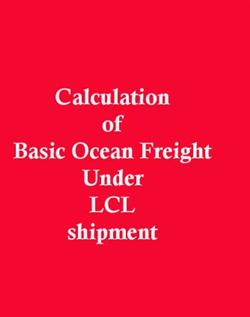## How to find Chargeable Volume?How to calculate Chargeable Volume?

Explain to find chargeable volume with examples under LCL export

Calculation of basic Ocean Freight under LCL cargo is on the basis of per CBM (Cubic Meter) rate or per Ton (per 1000 kg’s gross weight) whichever is higher.

Or it can be also stated as, basic ocean freight under LCL shipment is calculated on the basis of chargeable volume of cargo.

How to calculate chargeable volume of cargo?  Chargeable volume means total cubic meter volume of cargo or total gross weight in Tons whichever is higher.

More examples in which ‘Gross volume’ is accounted, for finding chargeable volume to calculate ocean freight.

Example 1

Find total ocean freight for the following LCL shipment. Freight charge is given by \$30 per CBM. There are 2 parcels and each parcel measuring

Length        - 3m

Width         -2m

Height        -80cm

Weight       - 3 tons

Solution

Volume            =length*weight*height

=3*2*0.8

=4.8 cbm

à 4.8*2 = 9.6 CBM

3 tons  = 3 CBM (1 tons= 1 CBM)

Basic Ocean Freight under LCL cargo is calculated on the basis of per CBM (Cubic Meter) rate or per Ton (per 1000 kgs gross weight) whichever is higher.

So,           9.6 > 3

i.e,                 =9.6*30

=\$288

Total ocean freight for the LCL shipment =\$288

Gross volume is accounted

Example 2:

Find total ocean freight for the following LCL shipment. Freight charge is \$42per cbm and gross weight is Weight - 3300kgs. The measurement of cargo is as follows;

Length – 65 cm ( i.e, 0.65 m)

Width - 2.5meter

Height - 3.2meter

Solution:

V       =  l*b*h

= 0.65*2.5*3.2

= 5.2cbm

Since 3300 is considered as 3.3 cbm (1000 kg = 1 cbm)

Basic Ocean Freight under LCL cargo is calculated on the basis of per CBM (Cubic Meter) rate or per Ton (per 1000 kgs gross weight) whichever is higher. So,

5.2 > 3.3

i.e,   5.2*42 = 218.4

Total ocean freight for the LCL shipment= \$218.4

Gross volume is accounted

Example 3:

Find ocean freight for the following LCL shipment.  Freight charge is 30\$per cbm ,total 3parcel measuring 3.5meter length,2.2meter width,90cm height respectively. Total weight is 15 tones.

Solution:

Length – 3.5m

Width  - 2.2 m

Height – 90 Cm i.e, 0.9 m

Parcel - 3

V        =  l*b*h

= 3.5*2.2*0.9

=6.93 cbm

i.e,     6.93*3

=20.79 cbm

Weight is 15 tons which is considered as 15 cbm, so when comparing

Basic Ocean Freight under LCL cargo is calculated on the basis of per CBM (Cubic Meter) rate or per Ton (per 1000 kgs gross weight) whichever is higher. So,

20.79 >15

i.e,       20.79*30 = 623.7

Total ocean freight for the LCL shipment = \$623.7

Gross volume is accounted

How to Choose a product for export business

How to get Export Orders?

How to settle dispute in Exports and Imports?

Pre shipment bank finance to suppliers for exports through other agencies

Types of export containers

How to import to India from Haiti?

How to export from India to Haiti?

How to export from El Salvador?

How to Import to El Salvador?

How to import to India from El Salvador?

How to export from India to El Salvador?

How to calculate IGST under imports?

Changes in IEC after GST

Linking IEC with GSTIN in customs

Import under EPCG after GST

GST on imports by EOUs and SEZs

Changes of operation of SEZ

Does CVD exists under project imports?

Baggage clearance after GST implementation

Meaning of Interstate dealer and seller

Inter State B2C Supply Means

Meaning of term Inter-State Goods and Service Tax (IGST) under GST

Inter and Intra State Level tax under GST

What is Integrated Tax under GST

Meaning of term Inspection and Search under GST

Seized Goods under GST